# 前端自动化测试详解（上）## 2 什么是自动化测试## 3 实施自动化测试有什么好处

• 可以验证代码正确性，保证项目质量
• 测试用例可以复用，一次编写，多次运行
• 通过看测试用例可以快速了解需求
• 驱动开发，指导设计，保证写的代码可测试

• 需求变动不频繁
• 项目周期足够长
• 自动化测试脚本可重复使用
• 代码规范可测试

## 5 自动化测试怎么做

### 5.1 原始的测试方法

1const sum = (a, b) => { return a + b }

1// test/util.test.js2const sum = (a, b) => { return a + b }3if(sum(1,1)===2){4    console.log('sum(1,1)===2, 测试结果符合预期，方法正确')5}else{6    console.log('sum(1,1)===2, 测试结果不符合预期，方法出错')7}1const sum = (a, b) => { return a + b + 1 }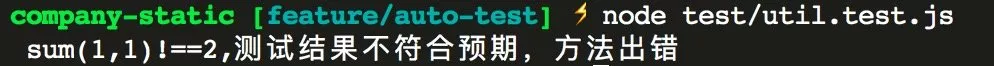1// test/util.test.js2const sum = (a, b) => { return a + b + 1 }3if (sum(1, 1) === 2) {4  console.log('    sum(1,1)===2, 测试结果符合预期，方法正确')5} else {6  throw new Error('sum(1,1)===2, 测试结果不符合预期，方法出错')7}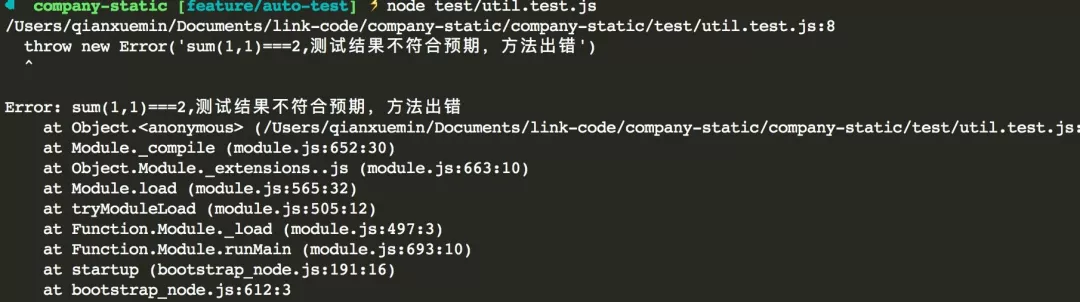1const sum = (a, b) => { return a + b + 1 }2const assert = require('assert')3assert.equal(sum(1, 1), 2)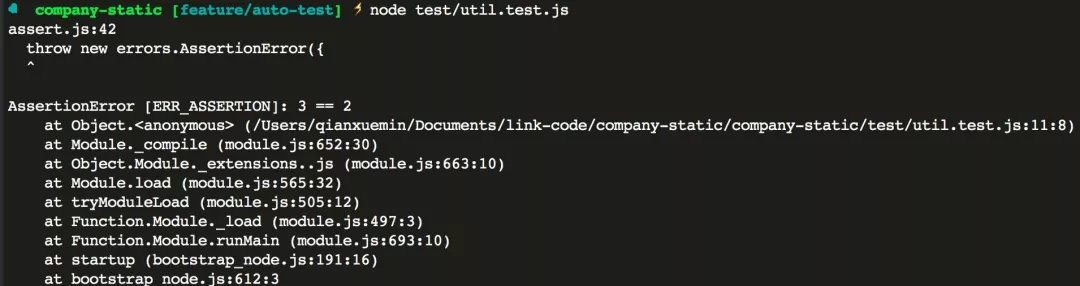assert 测试

### 5.2 使用测试框架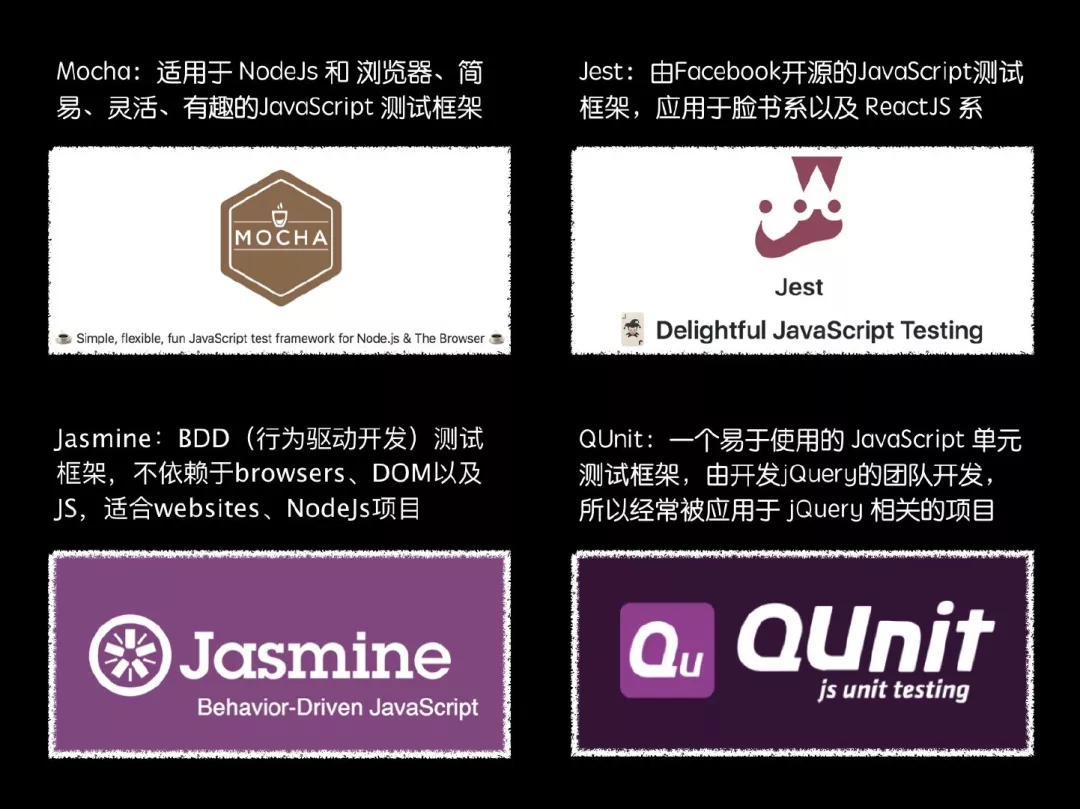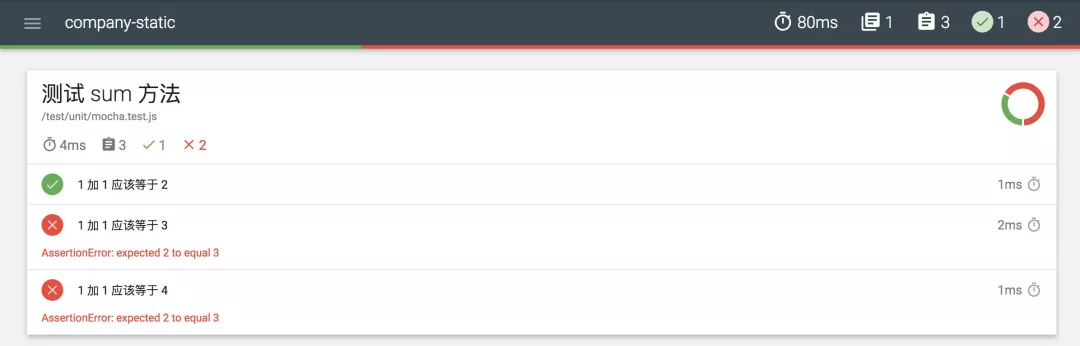html 格式的文档输出

### 5.3 测试方案技术选型

• 测试框架：mocha
• 断言库：chai
• 测试报告：mochawesome
• 测试覆盖率：Istanbul
• 测试浏览器：chrome
• 浏览器驱动：selenium-webdriver/chrome
• 接口测试 http 请求断言：supertest
• react 组件测试：enzyme
• 基准测试：benchmark

• 精简而灵活，扩展性强
• 社区成熟用的人多
• 各种测试用例在社区都能找到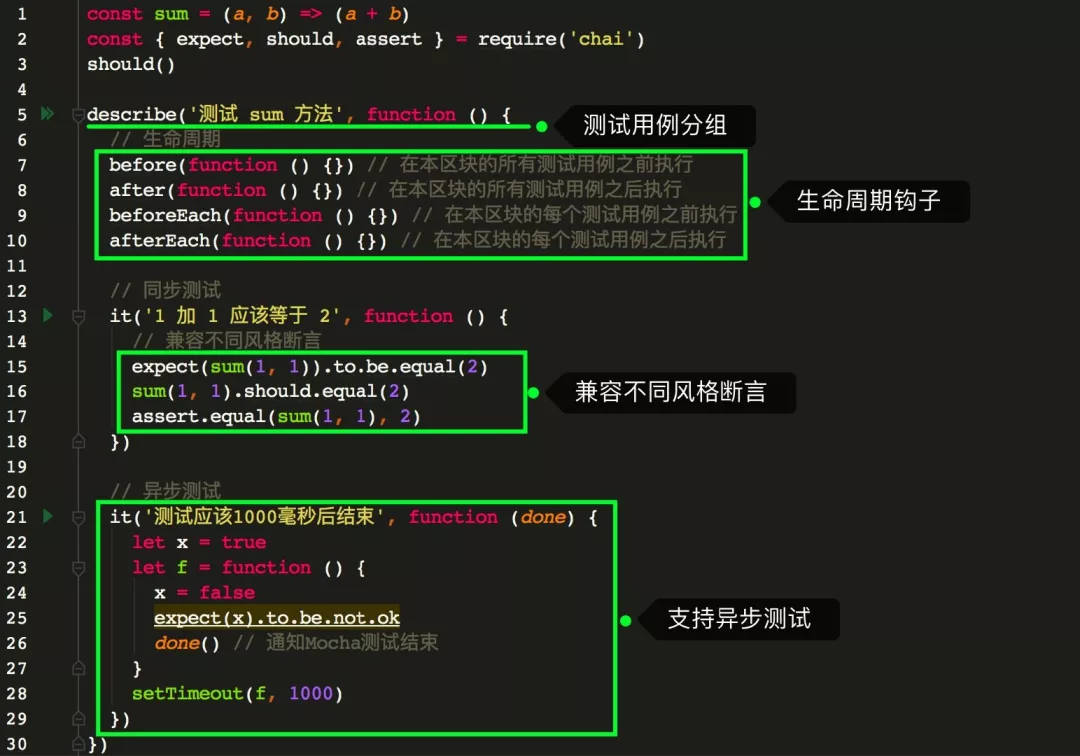Mocha 的能力

• 测试用例分组
• 生命周期钩子
• 兼容不同风格断言
• 同步异步测试架构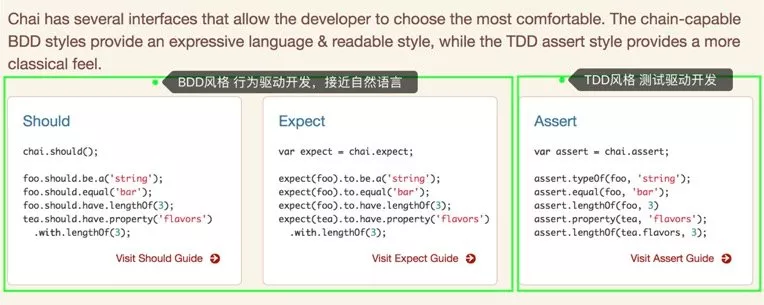chai 断言示例

## 评论2019 年 10 月 10 日 20:23